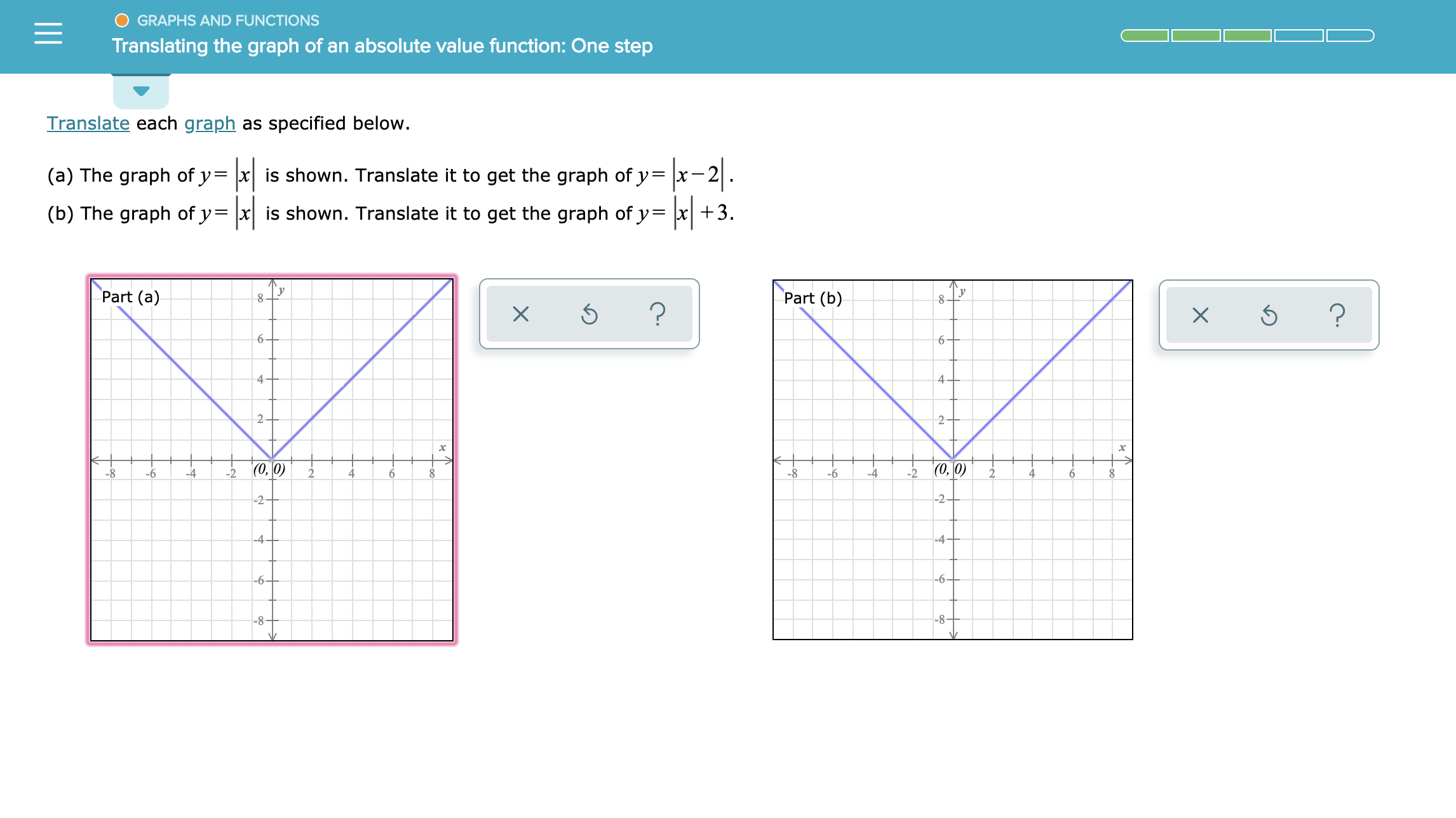# GRAPHS AND FUNCTIONSTranslating the graph of an ttsolute value function: One stepTranslate each graph as specified belowis shown. Translate it to get the graph of y= \x-.(a) The graph of y+3(b) The graph of y= |x| is shown. Translate it to get the graph of y=Part (a)Part (b)8??66-4+42|(0, 0)(0, 0)-22-8-4-224-8-4-6-64-2+-2-4+-4-66-8-

Question

See attachedhelp_outlineImage TranscriptioncloseGRAPHS AND FUNCTIONS Translating the graph of an ttsolute value function: One step Translate each graph as specified below is shown. Translate it to get the graph of y= \x-. (a) The graph of y +3 (b) The graph of y= |x| is shown. Translate it to get the graph of y = Part (a) Part (b) 8 ? ? 6 6- 4+ 4 2 |(0, 0) (0, 0) -2 2 -8 -4 -2 2 4 -8 -4 -6 -6 4 -2+ -2 -4+ -4 -6 6 -8- fullscreen
check_circleExpert Solution
Step 1
1. Translate the graph y=|x| to graph y = |x-2|
2. Translate the graph y = |x| to graph y=|x|+3
Step 2

The graph of the modulus function y= |x|

Step 3

The graph of the modulus function y = |x-2| shifts the graph y= |x| -2 units in positive x-axis

...

### Want to see the full answer?

See Solution

#### Want to see this answer and more?

Solutions are written by subject experts who are available 24/7. Questions are typically answered within 1 hour*

See Solution
*Response times may vary by subject and question
Tagged in

### Calculus